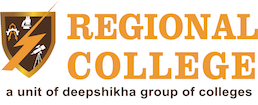# Application of Matrices

Matrices are a two-dimensional arrangement of numbers in row and column enclosed by a pair of square brackets or we can say matrices are rectangular arrangement of numbers, expression, symbols which are arranged in column and rows. According to Private Technical College in Rajasthan, Matrices have many applications in scientific field and practical real-life problem. Matrices can be applied in the study of electrical circuits, quantum mechanics and optics. Moreover, with the help of matrices, calculation of battery power outputs, resistor conversion of electrical energy into another useful energy can be done. The matrices play a role in calculation, with the help of matrices problem related to Kirchhoff law of voltage and current can be easily solved.

The most important usage of matrices in computer application is the encryption of message codes with the help of encryptions only; internal function is working and even could work with transmission of sensitive and private data. Best Private Engineering College in Rajasthan provide classes on matrices, which is a part of Engineering Mathematics. Matrices used in Statistics plays a vital role in scientific studies. Matrices are also used in robotics and automation in terms of base elements for the robot movements.

Areas of application-

One of the areas of computer science in which matrix multiplication is particularly useful is graphics, since a digital image is basically a matrix to begin with. The rows and columns of the matrix correspond to rows and columns of pixels, and the numerical entries correspond to the pixels’ colour values.

Matrix Multiplication can help to process digital sound. A digital audio signal is basically a sequence of numbers, representing the variation over time of the air pressure of an acoustic audio signal.
In this context, a graph is a mathematical construct consisting of nodes, usually depicted as circles, and edges, usually depicted as lines between them. Network diagrams and family trees are familiar examples of graphs, but in computer science they are used to represent everything from performed during the execution of a computer program to the relationships characteristic of logistics problems.

According to best btech college in Jaipur, every graph can be represented as a matrix, however, where each column and each row represent a node, and the value at their intersection represents the strength of the connection between them. Often, the most efficient way to analyse graphs is to convert them to matrices first.

### Alumni Panel

Nobody is bothered about an institution more than its alumni.